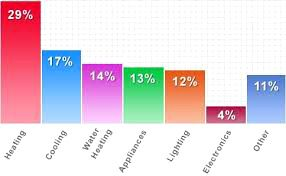# Statistics Quiz-4

0

Statistics Quiz-4Statistics Quiz-4

## Statistics Quiz-4

Question: Who invented the Lorenz line?

Answer: Dr Lorenz, a statistician, invented the Lorenz Line.

Question: What is meant by the Quaradic deviation?

Answer: The difference between one-third and one-fourth of the values ​​of the given data is called the inter-quadratic range and half of that difference is called ‘quadratic deviation’.

Question: What is meant by ‘Authentic Deviation?

Answer: The square of the parallel medium of the given information is called ‘authentic deviation’.

Question: Mention the formula for measuring the circumference.

Answer: The formula for measuring the circumference is–

R = L – S

where R = expansion

L = maximum value

S = smallest value.

Question: What is meant by correlation?

Answer: Correlation is a statistical method by which the variability between two or more variables can be studied.

Question: What is meant by positive correlation?

Answer: If two variables move in the same direction at the same time, then such correlation is called ‘positive correlation’.

Question: What is meant by negative correlation?

Answer: When two variables move in opposite directions, the correlation is called a negative correlation.

Question: What are the types of correlation?

Answer: There are three types of correlation, such as– positive, negative and zero correlation.

Question: If the change of two variables is different, then what kind of correlation will there be between the two variables?

Question: Does zero correlation mean uniqueness?

Question: When is the position correlation more accurate than the normal correlation coefficient?

Answer: When the variables cannot be calculated correctly, the correlation coefficient is more accurate than the normal correlation coefficient.

Question: What is an index?

Answer: The sum of statistical tools used to measure the change in the value of the interrelated variables is called ‘index’.

Q: What are the types of indices?

Q: What are the methods of index formation?

Answer: There are two methods of index formation: e.g. aggregate method and relative average method.

Question: What is the relative importance index of content?

Question: What are the weights in most indices?

Question: What index is used in general inflation?

Question: What is the most weighted item in the consumer price index of an industrial worker?